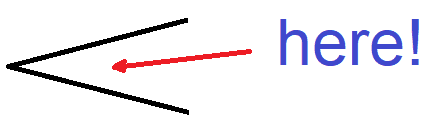# Interesting angles between 10~~25º ?

• I
• Nik_2213

#### Nik_2213

TL;DR Summary
Just as there are 'interesting' numbers, per primes, Pythag' triples etc, are there any 'interesting' angles between 10~~25º ??
NOT a home-work / college question, merely wondering...

Just as there are 'interesting' numbers, per primes, Pythag' triples, Euler-stuff etc, are there any 'interesting' angles between 10~~25º ??

I've had a hunt around, noticed several possibilities invoking eg transcendental fractions of radians etc, but...

Ideas ??

Combinations starting with 45 and 30 might be of interest.

are there any 'interesting' angles between 10~~25º ??
Define "interesting"

Define "interesting"
inter esse, lat. being between••Astronuc, mfb, jedishrfu and 2 others
Irrational angles might be interesting.

Last edited:
•jedishrfu
12 = 60/5 ~ pi/15
15 = 60/4 ~ pi/12
20 = 60/3 ~ pi/9
22.5 = 90/4 ~ pi/8

•bob012345
Irrational angles might be interesting.
Almost all angles are irrational.

•bob012345
Almost all angles are irrational.
It might be interesting that one can make by construction irrational angles and line segments.

How about: An angle is called interesting iff its sine is in a Galois extension of ##\mathbb{Q}.##

How about: An angle is called interesting iff its sine is in a Galois extension of ##\mathbb{Q}.##
Sorry, I dozed off there. What were you saying again?

Sorry, I dozed off there. What were you saying again?
That we can draw the darn thing with compass and ruler.

••malawi_glenn, pbuk and jbriggs444